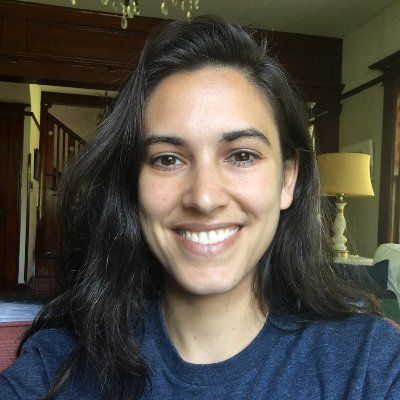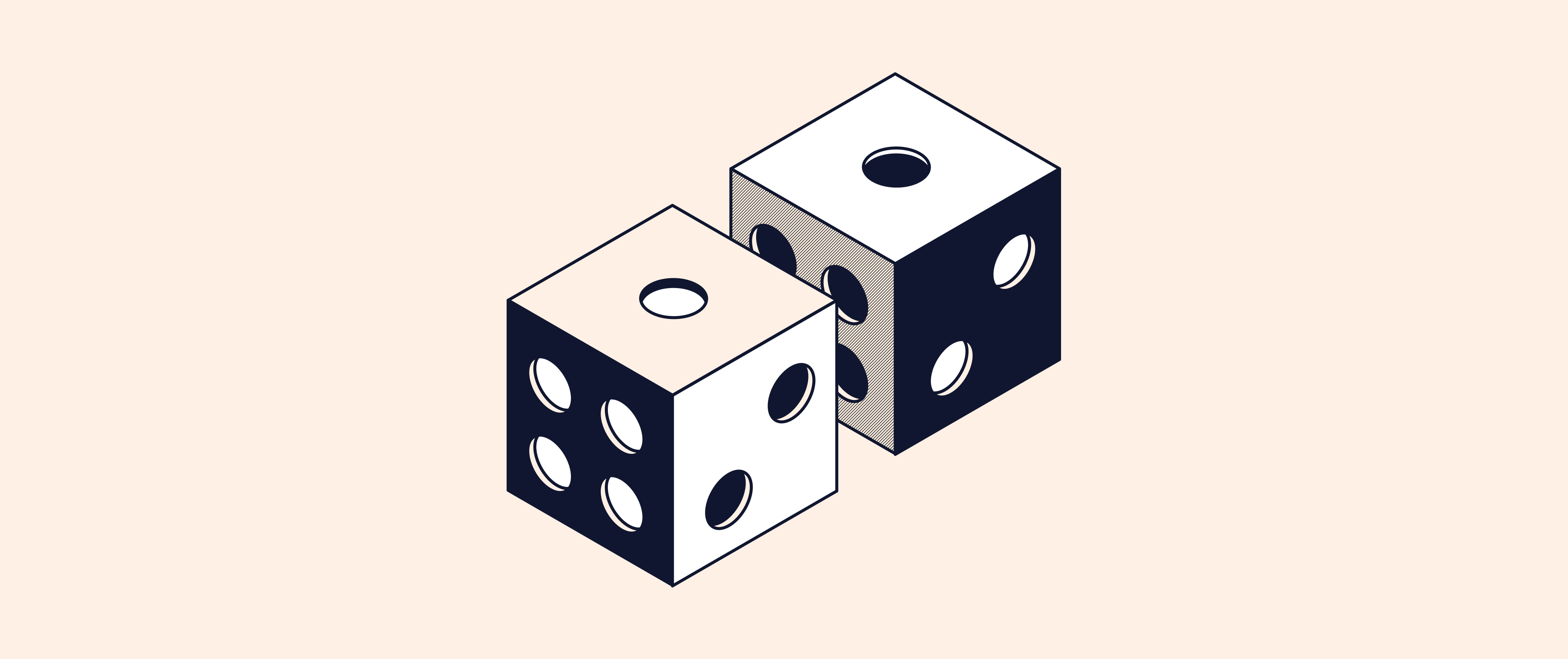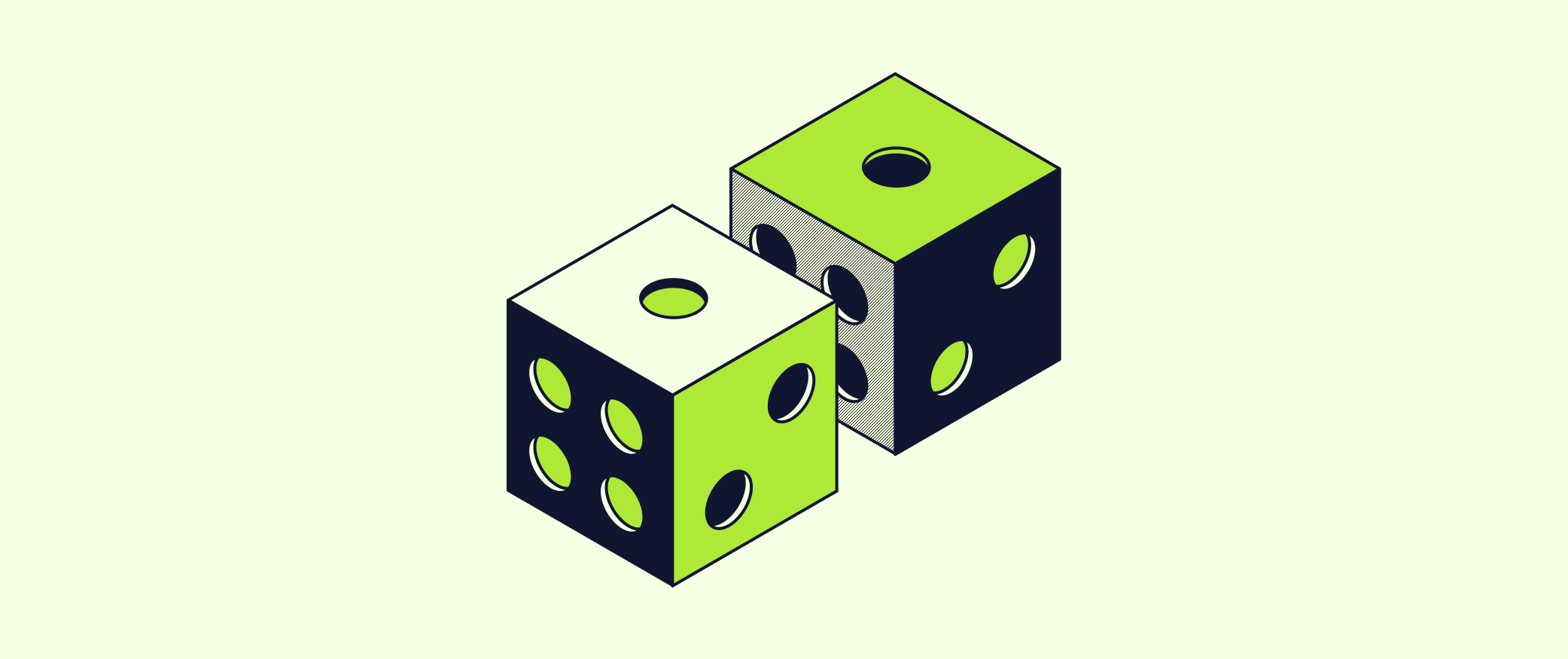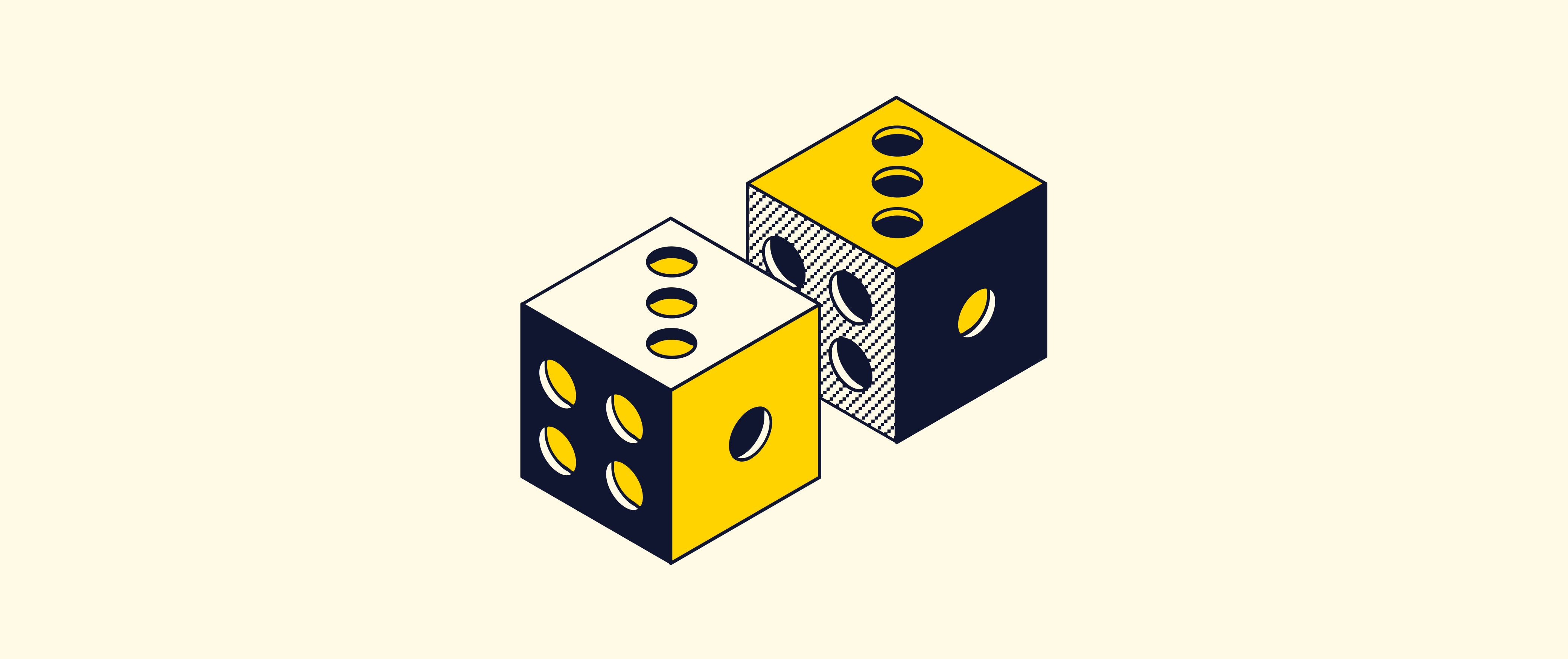# Level Up: Mastering statistics with Python - part 5

Rather than dig into complex math or over-simplify by using a pre-written function, we'll write our own binomial test function, primarily using base Python. In the process, we'll learn more about how hypothesis testing works and build intuition for how to interpret a p-value.,# Level Up: Mastering statistics with Python - part 4

While many introductory statistics classes teach the CLT, very few actually attempt to prove it because that requires some complex math. In this session, we'll bypass all that math by using Python loops to simulate the CLT.,# Level Up: Mastering statistics with Python - part 3

Comparing summary statistics like the mean and median can help us understand how these variables are related, but we can learn even more by using visualizations.,# Level Up: Mastering statistics with Python - part 2

Investigate a dataset with summary statistics and some basic data visualizations using the Python libraries NumPy, pandas, matplotlib, and Seaborn.,# Level Up: Mastering statistics with Python

In today’s tech industry, statistics and data science are becoming increasingly important and valuable skills.